# Math in Focus Grade 3 Chapter 3 Practice 3 Answer Key Addition with Regrouping in Ones, Tens, and Hundreds

Go through the Math in Focus Grade 3 Workbook Answer Key Chapter 3 Practice 3 Addition with Regrouping in Ones, Tens, and Hundreds to finish your assignments.

## Math in Focus Grade 3 Chapter 3 Practice 3 Answer Key Addition with Regrouping in Ones, Tens, and Hundreds

Question 1.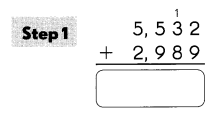Add the ones and regroup the ones.
2 ones + 9 ones
= ___ ones
= ___ tens ___ ones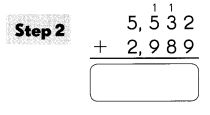Add the tens and regroup the tens.
1 ten + 3 ten + 8 tens
= ___ tens
= ___ hundred ___ tens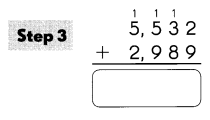Add the hundreds and regroup the hundreds.
1 hundred + 5 hundreds + 9 hundreds
= ___ hundreds
= ___ thousand ___ hundreds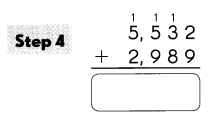1 thousand + 5 thousands + 2 thousands
= ___ thousands
5,532 + 2,989 = 8,521.

Explanation: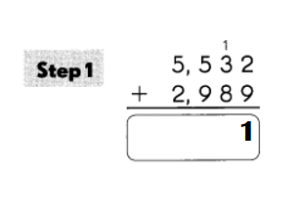Add the ones and regroup the ones.
2 ones + 9 ones
= 11 ones
= 1 tens 1 ones.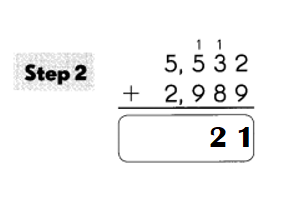Add the tens and regroup the tens.
1 ten + 3 ten + 8 tens
= 12 tens
= 1 hundred 2 tens.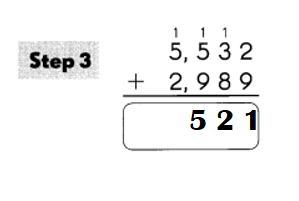Add the hundreds and regroup the hundreds.
1 hundred + 5 hundreds + 9 hundreds
= 15 hundreds
= 1 thousand 5 hundreds.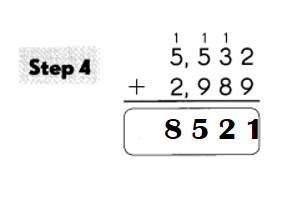1 thousand + 5 thousands + 2 thousands
= 8 thousands.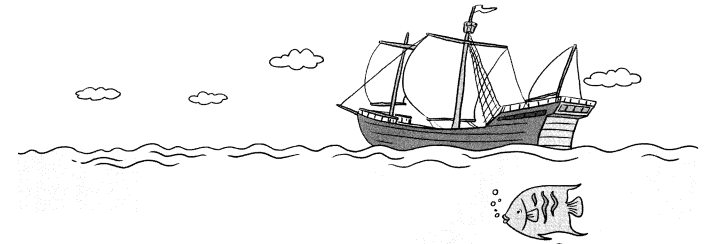Example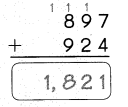Question 2.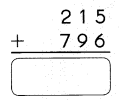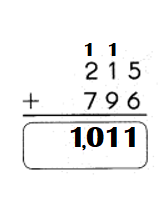Explanation:
215 + 796 = 1,011.

Question 3.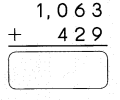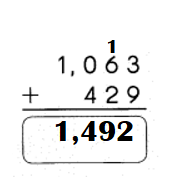Explanation:
1,063 + 429 = 1,492.

Question 4.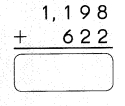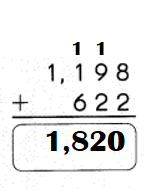Explanation:
1,198 + 622 = 1,820.

Question 5.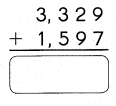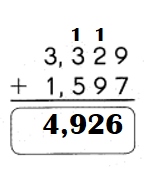Explanation:
3,329 + 1,597 = 4,926.

Question 6.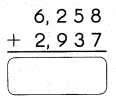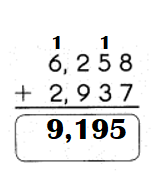Explanation:
6,258 + 2,937 = 9,195.

Question 7.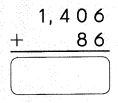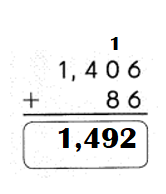Explanation:
1,406 + 86 = 1,492.

Question 8.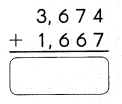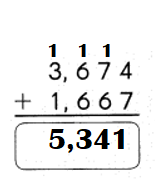Explanation:
3,674 + 1,667 = 5,341.

Question 9.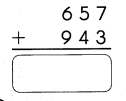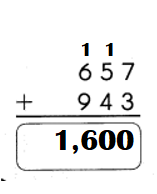Explanation:
657 + 943 = 1,600.

Question 10.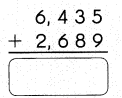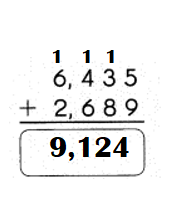Explanation:
6,435 + 2,689 = 9,124.

Fill in the blank.
Question 11.
Christopher Columbus landed on an island ¡n the Caribbean in _____
Christopher Columbus landed on an island ¡n the Caribbean in  1,492.

Explanation:Solve.
Example
A baker makes 3,452 bagels in the morning.
He makes another 759 bagels in the afternoon.
How many bagels does he make in all?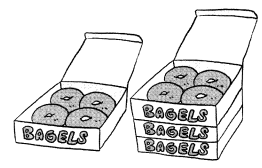3,452 + 759 = 4,211
The baker makes 4,211 bagels in all.

Question 12.
A grocer sells 6,835 apples and 2,795 oranges. How many pieces of fruit does she sell in all?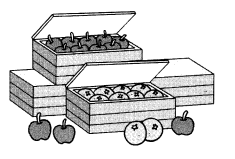She sells ___ pieces of fruit in all.
She sells 9,630 pieces of fruit in all.

Explanation:
Number of apples a grocer sells = 6,835.
Number of oranges a grocer sells = 2,795.
Total number of pieces of fruit she sells in all = Number of apples a grocer sells + Number of oranges a grocer sells
= 6,835 + 2,795
= 9,630.

Solve.
Question 13.
Molly’s bakery sells 5,268 muffins. Then Molly has 1,952 muffins left. How many muffins does she have at first?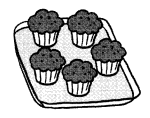Molly has ___ muffins at first.
Molly has 3,316 muffins at first.

Explanation:
Number of muffins Molly’s bakery sells = 5,268.
Number of muffins Molly has left = 1,952.
Number of muffins she have at first = Number of muffins Molly’s bakery sells – Number of muffins Molly has left
= 5,268 – 1,952
= 3,316.

Question 14.
Mr. Sanchez has 2,156 gold coins and 3,152 silver coins. How many coins does he have in all?
Mr. Sanchez has __ coins in all.